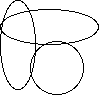Ever since a beginning course in point set topology I have wondered how to tell a 1D Euclidian space from a 2D space—ℝ from ℝ2. 52 years later I think I know how. It is embarrassingly simple.

(It turns out that Lebesgue had an even better and more general scheme since called ‘Lebesgue Covering Dimension’.)

The stuff below has no mathematical merit over the Wikipedia article. That article has a wonderful note in the talk section demonstrating why a simpler definition fails, and it is important to understand this failure.

The challenge is to say something, with predicate calculus and the predicate “open”, about the open sets of ℝ2 that is not so for those of ℝ. This is far short of characterizing the real line or square.

Let x, y and z range over point sets and “Ox” mean that x is open. Let “Cx” mean that x is connected and open.
Cx ↔ Ox∧~(∃y∃z (Oy∧Oz∧y≠∅∧z≠∅∧x=y∪z∧∅=y∩z))
(An open set is connected iff it is not the disjoint union of two non empty open sets.)

If n>1 and the topology is that of ℝn then there are three connected sets each pair of which intersect but the intersection of the three is empty.
∃x∃y∃z(Cx∧Cy∧Cz∧ x∩y≠∅ ∧ x∩z≠∅ ∧ y∩x≠∅ ∧ x∩y∩z=∅)It seems that connected sets in ℝ are intervals for which this arrangement is impossible. I suspect it is harder to tell the difference between ℝ2 and ℝ3. (2012, not really. See this for a transcription of “T is 2D” in pure predicate calculus.)

The one point compactification of ℝ is like ℝ2 in this regard since the set {x|x2>1}∪{∞} is connected there.The 4 sets above seem to be impossible on the circle however—intersecting by twos, disjoint by threes.

This does not characterize the line segment or circle; I was taught that that required the development of the reals. Yet a line segment might be characterized as a complete (in the sense of Dedekind) simple ordering without too many points. I suppose that that is already too much like the reals and still too loose. This may succeed in discriminating S(2) and S(3).